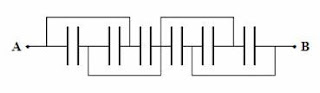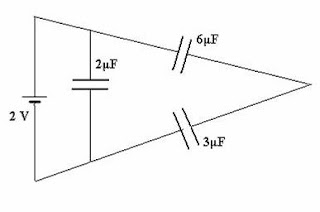## Sunday, July 31, 2011

### Kerala Engineering Entrance (KEAM -Engineering) 2011 Questions (MCQ) on Transistor Amplifier and Oscillator

Today we will discuss two multiple choice questions involving transistors which appeared in Kerala Engineering Entrance (KEAM -Engineering) 2011 question paper. These questions are simple as is the case with questions in this section at the level expected in the case of 12th grade (Plus Two) students.
(1) In a common emitter transistor amplifier, the output resistance is 500 KΩ and the current gain β = 49. If the power gain of the amplifier is 5×106, the input resistance is
(A) 325 Ω
(B) 165 Ω
(C) 198 Ω
(D) 225 Ω
(E) 240 Ω
Power gain Ap is given by
Ap = βac Av where βac is the current gain and Av is the voltage gain (for small a.c. signals).
Since Av = βacRo/Ri where Ro is the output resistance and Ri is the input resistance, we have
Ap = βac2Ro/Ri
Therefore Ri = βac2Ro/Ap = 492×(500×103) /(5×106) = 240 Ω, very nearly.
(2) A transistor oscillator is (i) an amplifier with positive feedback (ii) an amplifier with reduced gain (iii) the one in which dc supply energy is converted into ac output energy. Then
(A) all (i), (ii) and (iii) are correct
(B) only (i) and (ii) are correct
(C) only (i) and (iii) are correct
(D) only (ii) and (iii) are correct
(E) only (ii) is correct
Statements (i) and (iii) are correct where as statement (ii) is incorect. So the correct option is (c).

## Wednesday, July 20, 2011

### Multiple Choice Questions on Magnetic Force on Moving Charges

Questions involving magnetic force on moving charges are included in most of the medical, engineering and other degree entrance examinations. Here are some simple questions which may easily tempt you to commit mistakes:

(1) The magnetic Lorentz force equation is F = q v×B. In this equation

(a) F, v and B must be mutually perpendicular.

(b) F must be perpendicular to v but not necessarily to B.

(c) F must be perpendicular to B but not necessarily to v.

(d) v must be perpendicular to both F and B

(e) F must be perpendicular to both B and v.

The equation F = q v×B gives the magnetic force F on a charge ‘q’ when it moves with velocity v in a magnetic field B. The angle between the velocity v and the field B can be any value, but the magnetic force F is at right angles to both v and B. So the correct option is (e).

[The vector product v×B which gives the force F indeed demands that F is at right angles to both v and B].

(2) A charged particle moving in the north east direction at right angles to a magnetic field experiences a force vertically upwards. This charged particle will not experience any force if it moves towards(a) south west direction

(b) north

(c) east

(d) west

(e) north west

Since the magnetic force is vertical, the magnetic field must be horizontal.

Since the charged particle is moving in the north east direction at right angles to the magnetic field, it follows that the magnetic field must be directed either north west or south east (Fig.). So it will not experience any force if it moves towards north west or south east. The correct option is (e).

[You can use Fleming’s left hand rule to obtain the directions easily. See yourself that if the magnetic field in the above question is along the north west direction, the charge on the particle must be positive to obtain a vertically upward magnetic force. If the magnetic field in the above question is along the south east direction, the charge on the particle must be negative].

(3) A proton traveling vertically downwards experiences a southward force due to a magnetic field directed at right angles to its path.. An electron traveling northward in the same magnetic field will experience a magnetic force directed

(a) downwards

(b) upwards

(c) towards east

(d) towards west

(e) towards south east

Since the proton (which is positively charged) experiences a southward force while traveling vertically downwards, the perpendicular magnetic field must be acting towards the east.

[As required by Fleming’s left hand rule, hold the fore-finger, middle finger and thumb of your left hand in mutually perpendicular directions, with the middle finger pointing downwards (in the present case) and the thumb pointing southwards. The fore-finger then points towards the east].

If the proton were to move northward in this magnetic field, it would experience a downward magnetic force. Since the electron is negatively charged, it will experience an upward magnetic force [Option (b)]..

## Saturday, July 09, 2011

### Karnataka CET Multiple Choice Questions on Combination of Capacitors

The following question appeared in Karnataka Common Entrance Test (CET) 2010 question paper. Even though at the first reading it may appear somewhat difficult for you on seeing the circuit, a careful look at the circuit will assure you that it’s simple.All capacitors used in the diagram are identical and each is of capacitance C. Then the effective capacitance between the points A and B is

(a) 1.5 C

(b) 6 C

(c) C

(d) 3 C

The first three capacitors are in parallel, giving an effective capacitance 3 C. The last three capacitors also are in parallel, giving an effective capacitance 3 C.. These two parallel combinations are connected in series. Therefore, the effective capacitance between the points A and B is 3 C/2 = 1.5 C.

The following question appeared in the Karnataka CET 2008 question paper:

How many 6 μF, 200 V condensers are needed to make a condenser of 18 μF, 600 V?

(1) 9

(2) 18

(3) 3

(4) 27

Since the voltage rating of each 6 μF capacitor is 200 V, you should connect 3 capacitors in series to obtain the required voltage rating of 600 V. But then the effective capacitance of the series combination of these three capacitors will be 2 μF (6/3 = 2). To obtain the required capacitance of 18 μF, you need to connect nine such series combinations in parallel. So the total number of capacitors required is 9×3 = 27.The following question also appeared in the Karnataka CET 2008 question paper:

The total energy stored in the condenser system shown in the figure will be

(1) 2 μJ

(2) 4 μJ

(3) 8 μJ

(4) 16 μJ

The series combination of 6 μF and 3 μF gives an effective capacitance of (6×3)/(6+3) = 2 μF. Since this is in parallel with a 2 μF capacitor, the effective capacitance C across the 2 volt battery is 4 μF. The energy stored in the system of capacitors is ½ CV2 = (½)×(4×10–6) ×22 = 8×10–6 J = 8 μJ.

You will find a few more multiple choice questions (with solution) in this section here.